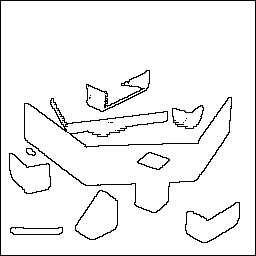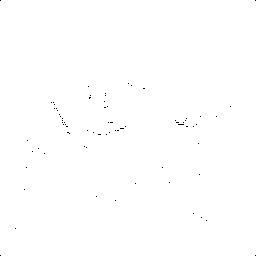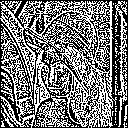PyCNN 0,2

Image Processing with Cellular Neural Networks in Python

PyCNN: Image Processing with Cellular Neural Networks in Python

Cellular Neural Networks (CNN) [wikipedia] [paper] are a parallel computing paradigm that was first proposed in 1988. Cellular neural networks are similar to neural networks, with the difference that communication is allowed only between neighboring units. Image Processing is one of its applications. CNN processors were designed to perform image processing; specifically, the original application of CNN processors was to perform real-time ultra-high frame-rate (>10,000 frame/s) processing unachievable by digital processors.

This python library is the implementation of CNN for the application of Image Processing.

Note: The library has been cited in the research published on Using Python and Julia for Efficient Implementation of Natural Computing and Complexity Related Algorithms, look for the reference #19 in the references section. I'm glad that this library could be of help to the community.

Note: Cellular neural network (CNN) must not be confused with completely different convolutional neural network (ConvNet).As shown in the above diagram, imagine a control system with a feedback loop. f(x) is the piece-wise linear sigmoid function. The control (template B) and the feedback (template A) templates (coefficients) are configurable and controls the output of the system. Significant research had been done in determining the templates for common image processing techniques, these templates are published in this Template Library.

Motivation

This is an extension of a demo at 14th Cellular Nanoscale Networks and Applications (CNNA) Conference 2014. I have written a blog post, available at Image Processing in CNN with Python on Raspberry Pi. The library was used in my paper B3: A plug-n-play internet enabled platform for real time image processing published in IEEE Xplore.

Dependencies

The library is supported for Python >= 2.7 and Python >= 3.3.

The python modules needed in order to use this library.

Pillow: 3.3.1
Scipy: 0.18.0
Numpy: 1.11.1 + mkl

Note: Scipy and Numpy can be installed on a Windows machines using binaries provided over here.

Example 1

\$ python example.py

OR

from pycnn import pycnn

cnn = pycnn()

Input:Edge Detection:

cnn.edgedetection('images/input1.bmp', 'images/output1.png')Grayscale Edge Detection

cnn.grayscaleedgedetection('images/input1.bmp', 'images/output2.png')Corner Detection:

cnn.cornerdetection('images/input1.bmp', 'images/output3.png')Diagonal line Detection:

cnn.diagonallinedetection('images/input1.bmp', 'images/output4.png')Inversion (Logic NOT):

cnn.inversion('images/input1.bmp', 'images/output5.png')Example 2

\$ python example_lenna.py

OR

from pycnn import pycnn

cnn = pycnn()

Input:Edge Detection:

cnn.edgedetection('images/lenna.gif', 'images/lenna_edge.png')Diagonal line Detection:

cnn.diagonallinedetection('images/lenna.gif', 'images/lenna_diagonal.png')Usage

Import module

from pycnn import pycnn

Initialize object

cnn = pycnn()

# object variables:
# m: width of the image (number of columns)
# n: height of image (number of rows)
# name: name of image processing method (say, Edge detection); type: string
# inputimagelocation: location of the input image; type: string.
# outputimagelocation: location of the output image; type: string.
# tempA_A: feedback template; type: n x n list, e.g. 3 x 3, 5 x 5.
# tempB_B: control template; type: n x n list, e.g. 3 x 3, 5 x 5.
# initialcondition: initial condition, type: float.
# Ib_b: bias, type: float.
# t: time points for integration, type: ndarray.
# Note: Some image processing methods might need more time point samples than default.
#       Display the output with each time point to see the evolution until the final convergence
#       to the output, looks pretty cool.

General image processing

cnn.generaltemplates(name, inputimagelocation, outputimagelocation, tempA_A, tempB_B,
initialcondition, Ib_b, t)

Edge detection

cnn.edgedetection(inputimagelocation, outputimagelocation)

Grayscale edge detection

cnn.grayscaleedgedetection(inputimagelocation, outputimagelocation)

Corner detection

cnn.cornerdetection(inputimagelocation, outputimagelocation)

Diagonal line detection

cnn.diagonallinedetection(inputimagelocation, outputimagelocation)

cnn.inversion(inputimagelocation, outputimagelocation)